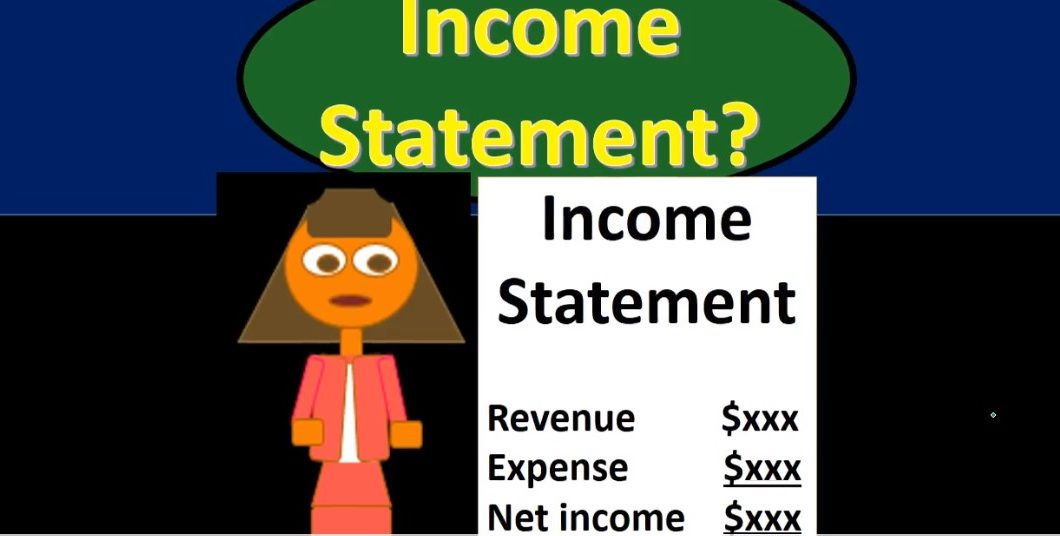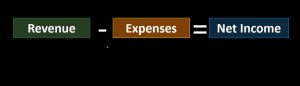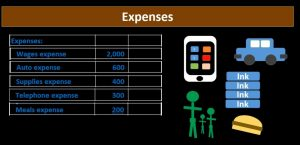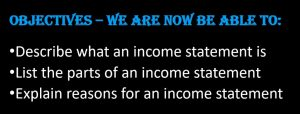## Income Statement 130

Hello in this presentation we will discuss the income statement objectives. At the end of this presentation, we will be able to describe what an income statement is list the parts of the income statement and explain the reasons for an income statement. First, we’ll start off with a question will which will explain the timing of the income statement or introduce us to an explanation of the timing of the income statement? And that is the question of asking somebody, how much do you make when we were to if we were to ask somebody how much they make, they would mentally make some type of assumption in order to answer that question, or they would ask you the question if they chose to answer at all.

00:43

The question, What do you mean, do you mean per month? Do you mean per year do you mean per week? And this is going to be something that needs to be answered in order to answer the question. Now oftentimes, if we were to ask somebody how much they make, they would make an assumption generally many times We’re going to say, Well, this is how much someone makes a year if we were to ask them and how much they make, they might just say, Oh, this is how much we make per year, or this is how much we make per month or per paycheck. But some type of assumption needs to be made in order for this question to be answered. Unlike a balance sheet type question.01:18

For example, if we were to ask somebody, how much money do they have? How much cash do they have? We don’t need to ask any other question. We don’t need to say what do you mean money per month. Now we just say as of this point in time, this is how much money we have as of now. So that’s going to be the primary difference between something that is an income statement related account and a balance sheet related account. The income statement being as of a time frame, having a beginning and an end. Those accounts revenue and expense needing a beginning and an end, a time frame such as a month, year or week, as opposed to those balance sheet accounts which are as of a point in time and don’t need any beginning and at the end but only one date. specifying a point in time.

02:02

For example, the date we are going to be looking at might be, or will be at this point, December 1 through December 31. That means that if we have revenue of 10,000, we’re talking about revenue that started at zero, and what’s counted upwards and during the time period of December December 1 through December 31, and is ending then at 10,000. In a similar way to if we were to drive a car and try to count how many miles we drove in a particular time frame, say a day, then we would say we started at zero, and we drove and increase the amount of miles that we drove during that day to 10,000 miles, we need to have that beginning point. And that ending point in order for that type of measurement to make sense, the measurement that we are measuring in terms of revenue is that type of measurement meant meaning it’s a measurement over a time.

02:56

So this is going to be the timeframe that will be represented on the Income Statement. And the income statement will represent that time frame, typically with wording such as for the month ended. So in this case, we’re going to say for the month end and note that the balance sheet is only going to have one date, it’s just going to say December 31 2000 x one, in this case, we don’t need for the month ended. We don’t need any other thing for that to make sense. It could be it doesn’t matter if if the rest of the financial statements were for the year or for the lifetime or whatnot in terms of the balance sheet is as of a point in time. But in terms of the income statement, we’re talking about something that started and ending a point in time.

03:37

Now note that typically, we will not see the starting point I don’t see December 1 on the income statement, we just see the ending date. And we see some phrase in this case for the month ended. If it was for the year ended, it would say for the year ended. And that phrase will of course indicate the fact that the starting point then is December 1. And of course the ending point is defined here, as December 31. When considering the income statement, we can think of the net income equation, that equation of revenue minus expenses equals net income. So the components of the income statement will be revenue and expense.

04:17

We may have some different types of income statements, some different subcategories, depending on the industry and the format of the income statement. However, the general the general categories will be the same meaning we’re going to have revenue accounts, we might have some subcategories of revenue, and we’re going to have expense accounts, we might have some subcategories of expenses. Right now we’re going to look at just a simplified income statement looking at those two components, not adding any subcategories at this point. Looking at basically what would typically be used for many service companies. When we move to merchandising companies and manufacturing companies. Then we often add some subcategories that will sub categorize some of the major most important expense and income relationships.04:59

So In this case, we’re going to say we’re do services. In terms of Computer Services. That’s how we’re going to get revenue. That’s what we do. And in order to generate revenue, note that most businesses, we’re only going to have like one or two main revenue accounts. Because when we think about specialization, in terms of a business, we are going to do what we do well concentrate on VAT in order to generate revenue resulting in a need for only one or two revenue accounts. And then we’re going to pay for everything else that we need to consume in order to help us to generate that revenue those being expenses. So in terms of amount of types of expenses, like the phone bill, the auto expense, not the auto itself, but the auto expense, wages, we might have supplies again we’re going to expense the supplies as we use the supplies although it’ll be an asset and then expensed and then we had might have meals and entertainment.

05:52

We’re gonna have all these different types of expenses and we’re gonna have a bunch more expenses typically. Then we have accounts for revenue however, With the hope is that the revenue account dollar amounts add up to more than the sum up of all the expense dollar amount accounts. So once again, in total, we will have more expense accounts typically. And we almost always we only have oftentimes one revenue main revenue account, but the revenue in dollar amount will be hopefully larger than the expense dollar amount and therefore we should have net income. And remember that net income is going to be part of the equity section. Therefore, we can kind of think of the net income as increasing the equity increasing the amount of that is going to go to the owner or owners or stockholders. In this case, we’re talking about an owner if we have a sole proprietor, there was a partnership then of course, the net income would increase what would then be allocated to the partners. And if we have a corporation, the net income would increase what would then be allocated to the shareholders.

06:57

Looking at the components of the income statement, we start Off with income or revenue. And in this case, we’re just going to say we do Computer Services. So we have a service company, we just do Computer Services. And that’s going to be the income. We’re going to say in our example that we had 10,000 of computer revenue for our example. And we’re just going to need one line item in order to report that on the income statement. Note that if we had two or more revenue sources, then we may put revenue as a subcategory, and then list those two revenue sources, and then put the those amounts in the inner column to be summed up in the outer column. However, if we only have one revenue source, then we may just put it in to the outer column here, because we don’t need any sub category.

07:41

So keep that in mind as we as we look and group things together. On the expense side of things, then, we typically will have more than one expense almost always, and therefore we will need a sub category that sub category being expenses represented by a colon. Then we’ll list the expenses and we will Typically indent the expenses and pull the amounts into an inner column, not representing debits and credits these two columns, but representing the fact that we’re going to have a subcategory in the inner column that will then be summed up in the outer column.

08:15

So here’s wages expense, we have auto expense, we have supplies expense, we have the telephone expense, and meals and entertainment, that’s going to be our last expense, we’re typically going to underline that, remember, again, that they are all indented here, then we’re going to sum up that subcategory and meaning we’re going to take the 2000 plus the 600, plus the 400, plus the 300, plus the 200, which will provide us with 3500. We’re going to indent once again total expenses and put that 3500 in this case in the outer column, not representing again debits or credits, but representing the subcategory of all the expenses then being summed up in the outer column also known That the order of the expenses is often a question as to what order should we put these expenses in.09:06

Now, it doesn’t have to be in any particular order. But we do want to put it in an order that will be best useful to the end users. Oftentimes, that order will be something like putting the biggest or largest expenses first. In this case, wages expense is often one of the bigger expenses for a company and then put it in descending order, many textbooks will actually will include a miscellaneous expense, and even if that’s a bigger amount will often put the miscellaneous expense on the bottom, because it’s kind of like our catch all category that doesn’t fit anywhere else. Note that if we sell inventory, then we’re clearly going to start generally with cost of goods sold, that being our most important and generally most large, the biggest expense as well.

09:51

So then we’re going to have our title on the income statement, which will include the name, the income statement, the name of the company, if we’re talking about an actual company, and Going to have the for the month ended. So remember, you’re always going to be asked that terminology, you always want to know what that terminology means, in that it’s going to differ from the balance sheet, the balance sheet is as of a point in time only showing a date, the income statement will be for a time frame, including a beginning date and an ending date. So we’re going to start off on the income statement with revenue revenue. Then, as we said, as we saw in our prior slide, that we have the 10,000 in the outer column from the computer revenue, then we have a subcategory of the expenses, listing all the expenses, and then the sub category being totaled in the outer column.

10:40

We didn’t have within the outer column, just revenue and expenses. What we will then do is subtract that out. So we’re going to subtract that out. How do we know it’s a subtraction problem? We just know that it’s so common. Well, that’s the idea. Typically, if we’re going to subtract something out, we won’t often put brackets around. Around the subtraction problem, so I’m not going to put a negative 3500 or a minus sign to indicate that we’re subtracting it.

11:07

Oftentimes in many other areas, we’ll actually put in the text that we’re going to say less, for example, less total expenses. However, the income statement because it’s such a common statement, it’s assumed to be known that we’re going to subtract revenue minus expenses. Also note that we don’t typically jump around from column to column, something that’s obvious here, but more important when we get to more complex financial statements, meaning when we calculate this 6500, we’re not we’re only taking this outer column, this 10,000 minus the 3005. We don’t typically go from the 3005 for example, and jump into this column and then jump back to this column, we will typically only add or subtract or perform some kind of mathematical operation on one column at a time, this time being the outer column.11:57

So the bottom line number then, of the income statement is Course net income. Now if the revenue was less than the expenses, then clearly we would have a net loss and this would be a you know, we’d have a loss here and we’d be losing money for the time period. Remember that this bottom line number is going to be an increase in the equity section when we consider the income statement, it is a part of the equity section in that revenue is increasing equity expenses are decreasing equity, equity will be going in the same direction as the net income is going. So whenever revenue goes up, equity goes up. Whenever expenses go up, equity goes down. When net income goes up, revenue, then then equity goes up. When net income goes down, then equity goes down. Recall once again that these are going to be the timing statements meaning this net income represents the net value of what we have earned over a certain period of time in this case, this being the month of December December 1 through December 31. This is the bottom line number of course of the income statement. This is how we did over that time period. And it only makes sense if we have those two dates if we have the beginning date and the ending date. objectives, we are now able to describe what an income statement is. list the parts of the income statement and explain reasons for an income statement.